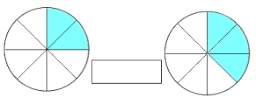Add this two mixed numbers: 1 5/6 + 2 2/11=

f =  4.0152

### Step-by-step explanation:

$f=1+\frac{5}{6}+\left(2+\frac{2}{11}\right)=\frac{265}{66}=4\frac{1}{66}=4.0152$Did you find an error or inaccuracy? Feel free to write us. Thank you!Tips to related online calculators
Need help to calculate sum, simplify or multiply fractions? Try our fraction calculator.

## Related math problems and questions:Add two mixed fractions: 2 4/6 + 1 3/6Add this two mixed numbers: 1 5/6 + 2 2/11=Why does 1 3/4 + 2 9/10 equal 4.65? How do you solve this?
• FractionsSort fractions z1 = (6)/(11); z2 = (10)/(21); z3 = (19)/(22) by its size. Result write as three serial numbers 1,2,3.Add marks (+, -, *, /, brackets) to fullfill equations 1 3 6 5 = 10 This is for the 4th grade of the primary school - with no negative numbers yet
• Two pizzasJacobs mom bought two whole pizzas. He ate 2/10 of the pizza and his dad ate 1 1/5. How much is left.
• Evaluate 11Multiply the quotient of 6 and 2 by 3, then add 1. Add 1 and 6, then divide by 2 and multiply by 3. Divide 6 by the product of 2 and 3, then add 1.What is 4 1/2+2/7-213/14?
• Mixed numbersRewrite mixed numbers, so the fractions have the same denominator: 5 1/5 - 2 2/3Add and write the result again as hours, minutes, seconds: 2hodiny45min15s + 1h20m50s =
• Expression 1What is 7+8-(5×2)+5-4+(6×(5-3)+6)-(8+10)-7+6?2 and 1/8th plus 1 and 1/3rd =(a) Convert the following mixed numbers to improper fractions. i. 3 5/8 ii. 7 7/6 (b) Convert the following improper fraction to a mixed number. i. 13/4 ii. 78/5 (c) Simplify these fractions to their lowest terms. i. 36/42 ii. 27/45 2. evaluate the follow3 3/4 + 2 3/5 + 5 1/2 Show your solution.Divide the product of 4 and 5/8 by 1 1/2. Write your answer as a mixed number.Father has 12 1/5 meters long wood. Then I cut the wood into two pieces. One part is 7 3/5 meters long. Calculate the length of the other wood?What is the sum of 2/3 and 3/10?